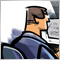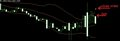# OrderClose is closing order OP_SELL above and OP_BUY bellow the OrderOpened ?22

I'm trying to create a dynamic Take Profit programatically. To do this I am using OrderClose() to close the order when the difference of pips are ready. The problem is that it is closing above the OP_SELL and bellow OP_BUY and I don't know why it happens.

how could I fix this ?

See the image: https://imgur.com/B0LYbkC

```void executeTakeProfit(int mn, double points){
double _diffPips = 0;

if(OrdersTotal() > 0){
RefreshRates();

for(int x = 0; x < OrdersTotal(); x++){
if(OrderMagicNumber() == mn && OrderSymbol() == Symbol()){

_diffPips = NormalizeDouble((Ask - OrderOpenPrice()) / Point, Digits);
if(_diffPips >= points){
if(!OrderClose(OrderTicket(), OrderLots(), Bid, 0, clrNONE)){
}
}
}

if(OrderType() == OP_SELL){
if(Bid < OrderOpenPrice()){
_diffPips = NormalizeDouble((OrderOpenPrice() - Bid) / Point, Digits);
if(_diffPips >= points){
Comment("Error Take Profit - Sell");
}
}
}
}//sell

}//magic

}//select
}//for

}

}//execute take profit
```Imgur
• 2020.01.11
• imgur.com
Post with 0 votes and 1 views.20223

Fernando Paiva: closing above the OP_SELL and bellow OP_BUY
```if(Ask > OrderOpenPrice()){
⋮
if(!OrderClose(OrderTicket(), OrderLots(), Bid, 0, clrNONE)){```

If the Ask goes one tick above the open price, the Bid (close price) is below the open price (by the spread.)

You buy at the Ask and sell at the Bid. So for buy orders you pay the spread on open. For sell orders you pay the spread on close.
1. Your buy order's TP/SL (or Sell Stop's/Sell Limit's entry) are triggered when the Bid reaches it. Not the Ask. Your SL is shorter by the spread and your TP is longer. Don't you want the same/specified amount for either direction?
MODE_SPREAD (Paul) - MQL4 programming forum - Page 3 #25
3. The charts show Bid prices only. Turn on the Ask line to see how big the spread is (Tools → Options (Control-O) → charts → Show ask line.)22

William Roeder:

If the Ask goes one tick above the open price, the Bid (close price) is below the open price (by the spread.)

Are you mean the problem is the spread ? what do you suggest in this case of my function ?8714

Fernando Paiva:

Are you mean the problem is the spread ? what do you suggest in this case of my function ?

```if(!OrderClose(OrderTicket(), OrderLots(), Bid, 0, clrNONE)){

OrderClosePrice()```

In your case here Bid is used, try the other function i provided ;)22

Kenneth Parling:

In your case here Bid is used, try the other function i provided ;)

I've tried to change the function but it still closing above at OP_SELL and bellow at OP_BUY. Could you help me ?

Trying

```void executeTakeProfit(int mn, double points){
double _diffPips = 0;

if(OrdersTotal() > 0){

for(int x = 0; x < OrdersTotal(); x++){
if(OrderMagicNumber() == mn && OrderSymbol() == Symbol()){

_diffPips = NormalizeDouble((OrderClosePrice() - OrderOpenPrice()) / _Point, Digits);
if(_diffPips >= points){
RefreshRates();
if(!OrderClose(OrderTicket(), OrderLots(), OrderClosePrice(), 0, clrNONE)){
Comment("Take Profit Error - Buy", GetLastError());
}
}

if(OrderType() == OP_SELL){
_diffPips = NormalizeDouble((OrderClosePrice() - OrderOpenPrice()) / _Point, Digits);
if(_diffPips <= points){
RefreshRates();
if(!OrderClose(OrderTicket(), OrderLots(), OrderClosePrice(), 0, clrNONE)){
Comment("Take Profit Error - Sell", GetLastError());
}
}
}//sell

}//magic

}//select
}//for

}

}//execute take profit```2259

Fernando Paiva:

I've tried to change the function but it still closing above at OP_SELL and bellow at OP_BUY. Could you help me ?

Trying

awkay now How On earth does an open order have a value for OrderClosePrice()

```f(OrderType() == OP_BUY){
_diffPips = NormalizeDouble((OrderClosePrice() - OrderOpenPrice()) / _Point, Digits);
if(_diffPips >= points){
RefreshRates();
if(!OrderClose(OrderTicket(), OrderLots(), OrderClosePrice(), 0, clrNONE)){
Comment("Take Profit Error - Buy", GetLastError());
}
}

if(OrderType() == OP_SELL){
_diffPips = NormalizeDouble((OrderClosePrice() - OrderOpenPrice()) / _Point, Digits);
if(_diffPips <= points){
RefreshRates();
if(!OrderClose(OrderTicket(), OrderLots(), OrderClosePrice(), 0, clrNONE)){
Comment("Take Profit Error - Sell", GetLastError());
}```

ok So we want a virtual Take Profit. lets do this

Have Holder of the Pips you want for your take Profit

`input int m_takeprofit = 40; //Take Profit in pips`

then next we need to change that into the corrct Format

```void executeTakeProfit(int mn, double points){
double _diffPips = 0;
double takeprof;

if(OrdersTotal() > 0){

for(int x = 0; x < OrdersTotal(); x++){
if(OrderMagicNumber() == mn && OrderSymbol() == Symbol()){
takeprof = m_takeprofit * 10 * SymbolInfoDouble(OrderSymbol(),SYMBOL_POINT);

_diffPips = NormalizeDouble((SymbolInfoDouble(OrderSymbol(),SYMBOL_BID) - OrderOpenPrice()) , SymbolInfoInteger(OrderSymbol(),SYMBOL_DIGITS));
if(_diffPips >= takeprof ){
RefreshRates();
if(!OrderClose(OrderTicket(), OrderLots(), OrderClosePrice(), 0, clrNONE)){
Comment("Take Profit Error - Buy", GetLastError());
}
}

if(OrderType() == OP_SELL){
_diffPips = NormalizeDouble((OrderOpenPrice() - SymbolInfoDouble(OrderSymbol(),SYMBOL_ASK)) , SymbolInfoInteger(OrderSymbol(),SYMBOL_DIGITS));
if(_diffPips >= takeprof ){
RefreshRates();
if(!OrderClose(OrderTicket(), OrderLots(), OrderClosePrice(), 0, clrNONE)){
Comment("Take Profit Error - Sell", GetLastError());
}
}
}//sell

}//magic

}//select
}//for

}

}//execute take profit```

I am not sure if there errors but u get the Idea heyModerator
20446

Jefferson Metha:

awkay now How On earth does an open order have a value for OrderClosePrice()

How does an open bar have a value for Close? (rhetorical question)

It's the same sort of thing.

OrderClosePrice() is the last known value at the time that the order is selected, so for a buy, it will be the same as Bid and for a sell, the same as Ask.2259

Keith Watford:

OrderClosePrice() is the last known value at the time that the order is selected, so for a buy, it will be the same as Bid and for a sell, the same as Ask.

What, it doesn't make sense to me, but I understand the Close example
I thought OrderclosePrice must be accessed by

 if(OrderSelect(i,SELECT_BY_POS,MODE_HISTORY)

I am having a BrainShutdown trying to process that. lemme go sleep its 3 am my time20223

You can use OrderClosePrice() instead of Bid/Ask and be direction independent — no need to check order type for close price. But if you potentially close multiple orders, you must call RefreshRates after the server call and before the next OrderSelect.